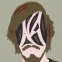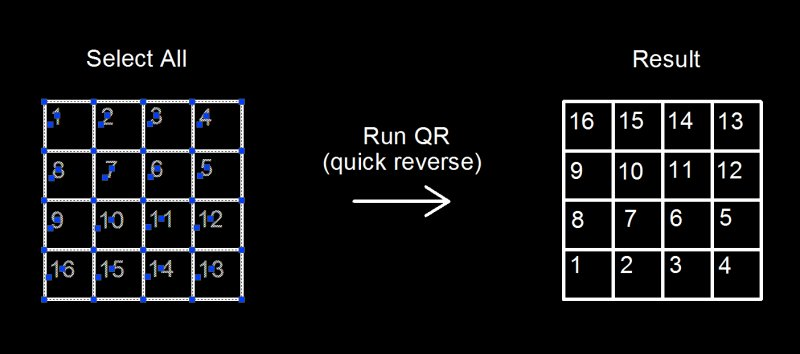# Reverse Numbers

## Recommended PostsI regularly use text to number objects for designating cut order. In the past, I have cobbled together some code that can quickly increment or decrement all selected numerical text in case I make a mistake and/or have to change something. This works marvelously, but often, I find that I need to go beyond that and completely reverse the order.

Using this code as a starting point, I thought this would be super simple but the fact that I'm trying to do list manipulation to text objects is completely throwing me off. I'm still a bit new to this sort of thing. I was thinking I'd get a list of all selected text, sort it, then reverse that list before applying changes to the text. Highest number gets swapped with the lowest, second highest with second lowest, etc.

("1" "2") would become ("2" "1")

("2" "1" "4" "5" "3") would become ("4" "5" "2" "1" "3")

("2" "8" "17" "4") would become ("17" "4" "2" "8")

("3" "5" "-4") would become ("3" "-4" "5")

I'm not sure where to start, nor what process to use. My main problem is that I can't figure out how to sort and edit the data without losing links to the correct text objects. Any help would be awesome. Here is the my quick increment/decrement routine that I'm trying to start with.

```;Quick Increment/decrement
;Increments/decrements all selected text integers by 1
;I hacked this code together with help from an "increment in range" routine.
;Original code can be found here https://forums.autodesk.com/t5/visual-lisp-autolisp-and-general/incriment-numbered-text/td-p/3614646?nobounce

;Quick Increment
(defun C:qi (/ textselection textobject old)
(setq textselection (ssget '((0 . "TEXT"))))
(repeat (sslength textselection)
(setq
textobject (vlax-ename->vla-object (ssname textselection 0))
old (vla-get-TextString textobject); initial text content
); setq
(if
(and
(= (strlen old) (strlen (itoa (atoi old)))); text represents integer
; Blocks e.g. "12A", "4.5", "C37", and completely non-numerical
;   text [except for next test], but allows negative integers.
; Also disallows e.g. "06", "008", so if those should be included...
(if (= (strlen old) 1) (wcmatch old "#") T)
; blocks single non-numerical character e.g. "A" [passes prior test]
); and
(vla-put-TextString textobject (itoa (+ (atoi old) 1))); replace
); if
(ssdel (ssname textselection 0) textselection)
); repeat
(princ)
(setq sel1 (ssget "P"))
(sssetfirst nil sel1)
); defun

;Quick decrement
(defun C:qd (/ textselection textobject old)
(setq textselection (ssget '((0 . "TEXT"))))
(repeat (sslength textselection)
(setq
textobject (vlax-ename->vla-object (ssname textselection 0))
old (vla-get-TextString textobject); initial text content
); setq
(if
(and
(= (strlen old) (strlen (itoa (atoi old)))); text represents integer
; Blocks e.g. "12A", "4.5", "C37", and completely non-numerical
;   text [except for next test], but allows negative integers.
; Also disallows e.g. "06", "008", so if those should be included...
(if (= (strlen old) 1) (wcmatch old "#") T)
; blocks single non-numerical character e.g. "A" [passes prior test]
); and
(vla-put-TextString textobject (itoa (- (atoi old) 1))); replace
); if
(ssdel (ssname textselection 0) textselection)
); repeat
(princ)
(setq sel1 (ssget "P"))
(sssetfirst nil sel1)
); defun```

##### Share on other sitesHi Benhubel. Here's few thougths

To sort strings, you could use the acad_strlsort function. The downside is that it will classify them using ascii values.

```(acad_strlsort '("4" "5" "2" "1" "3" "10"))
("1" [i]"10"[/i] "2" "3" "4" "5")```

If all the numbers you have are the same length, (01, 04, 12, 16), it will do the job "as is". If not you will need to manipulate things. Either you...

-Check for the digit with the biggest strlen, and add as many 0 in front of the digits , sort them and then remove the 0's after sorting. Painfull

-or you could retrieve the strings containing the integers, swap them to integers before creating the list to vl-sort it or

-just leave it as is (list of strings) and use atoi on each member, to rebuild a new list...

```(defun test ( lst / retlist)
(foreach mem lst
(setq retlist (cons (atoi mem) retlist))
)
(vl-sort retlist '<)
)```

(test '("4" "5" "002" "1" "3" "10"))

(1 2 3 4 5 10)

Boom!

Another thing I do if I need to keep a link with a said thing (lets say value and ), I create a list of dotted pairs instead of a plain list. ((value1 . )(value2 . )(etc..)) for instance. You can then vl-sort it by the car, and when it is time to handle the information later on, you can retrieve the corresponding easily with cdr. That should be a good starting point for you.

Hope it helps

Cheers

Edited by Jef!
typo fix

##### Share on other sitesAnother thought:

```_\$ (vl-sort '("4" "3" "10" "0" "0.52" "1" "-3.4" "-0.25" "5" "2" "-2" "3.5" "6.8")
'(lambda (a b) (apply '< (mapcar 'read (list a b))))
)
("-3.4" "-2" "-0.25" "0" "0.52" "1" "2" "3" "3.5" "4" "5" "6.8" "10")
_\$ ```

##### Share on other sites("1" "2") would become ("2" "1")

("2" "1" "4" "5" "3") would become ("4" "5" "2" "1" "3")

("2" "8" "17" "4") would become ("17" "4" "2" "8")

("3" "5" "-4") would become ("3" "-4" "5")

Hi benhubel

I don't know what the code you posted has anything to do with the quoted task. Here is a sample of how you could swap the min/max values in the list:

```(defun swap (l / r n)
(setq r (vl-sort l
'(lambda (a b)
(< (atoi a) (atoi b))
)
)
)
(setq n (1- (length l)))
(mapcar
'(lambda (x)
(nth (- n x) r)
)
(vl-sort-i
(vl-sort-i
(mapcar 'atoi l)
'<
)
'<
)
)
)```

```_\$ (swap '("1" "2")) -> ("2" "1")
_\$ (swap '("2" "1" "4" "5" "3")) -> ("4" "5" "2" "1" "3")
_\$ (swap '("2" "8" "17" "4")) -> ("17" "4" "2" "8")
_\$ (swap '("3" "5" "-4")) -> ("3" "-4" "5")```

About the number-entity association, please describe more precise what you are after.

You can make an associated list, as suggested by Jef, but is still unclear for me.

A list like

`(("3" . Ent1) ("1" . Ent2) ("2" . Ent3))`

would become

`(("1" . Ent1) ("3" . Ent2) ("2" . Ent3))`

or

`(("1" . Ent2) ("3" . Ent1) ("2" . Ent3))`

Note Ent1 and Ent2 initial and final position.

##### Share on other sitesThank you for the replies.

The code I quoted is my starting point for dealing with the text objects. I'm aiming for the finished program to work the same as code I posted, except that instead of incrementing the numbers, it reverses them.

I will experiment with the examples you guys gave and see what I can build. It looks like the associated list will probably be the way to go if I'm able to make it work correctly.

Just for clarity, this image is an example of what I am attempting to do.##### Share on other sitesNot necessary a assoc list. In fact, it is more easy in this case with 2 different lists.

```(defun c:qr ( / ss i e s obj num n u m)
(if
(setq ss (ssget "_:L" '((0 . "TEXT"))))
(progn
(repeat (setq i (sslength ss))
(setq e (vlax-ename->vla-object (ssname ss (setq i (1- i))))
s (vla-get-textstring e)
)
(if
(and (setq d (distof s)) (equal d (fix d) 1e-)
(setq obj (cons e obj)
num (cons (atoi s) num)
)
)
)
(if num
(progn
(setq n (vl-sort-i num '<)
u (mapcar '(lambda (x) (nth x num)) n)
m (1- (length num))
)
(mapcar
'(lambda (e x)
(vla-put-textstring e (itoa (nth (- m x) u)))
)
obj
(vl-sort-i n '<)
)
)
)
)
)
(princ)
)```

Works with duplicates, but the result is unpredictable (I guess it shouldn't be any duplicate).

##### Share on other sitesWoah, that works great! I'll have to look it over and see if I can understand everything that's going on in it. Thank you very much!

## Join the conversation

You can post now and register later. If you have an account, sign in now to post with your account.
Note: Your post will require moderator approval before it will be visible.×   Pasted as rich text.   Paste as plain text instead

Only 75 emoji are allowed.